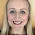## 14 November 2016

### New GCSE: Trigonometry Questions

I've been teaching trigonometry to my top set Year 11 over the last couple of weeks. The content is much the same as it was on the old GCSE but the exam questions may now be considerably more challenging.

The only new trigonometry content in the Higher tier is 'exact values' (ie non-calculator trigonometry), which requires sound knowledge of surds.

I wrote a blog post about Teaching Trigonometry a couple of years ago and I have loads of resources listed in my shape library so I won't repeat it all here, but I just wanted to mention a few interesting GCSE trigonometry questions that I found when looking through the questions collated by Mel (@Just_Maths) here and here. If you teach Year 11s who will be taking the higher tier exams then you might find these questions helpful in your lesson planning.

1. Ambiguous case
I always teach the ambiguous case at GCSE but I've not seen many exam questions on it before. It's interesting to see this three mark question in AQA's higher Specimen Paper 3.

At first glance it seems like a straightforward Sine Rule question but we're told that the angle is obtuse, meaning students have to subtract their initial answer from 180.

I've spotted a couple of questions in the specimen papers that refer to acute and obtuse angles so I do think it's important to teach the ambiguous case.

I recommend that you have a go at this question to see how challenging it would be for the vast majority of Year 11s.
It's from WJEC Eduqas's sample assessment materials (the last question on the higher non-calculator paper). It requires knowledge of the value of sin60 and cos60. It requires students to solve a quadratic equation and work with surds. They also have to recall the formulae for the Cosine Rule and area of a triangle using sine.

They get eight marks (and in my opinion deserve an automatic Grade 9!) if they get this question right.

3. Angles in parallel lines and similarity
Here's a four mark question from WJEC Eduqas's sample assessment materials.
There are two common approaches here: the first is to use angles facts to find the angles in triangle ABC, then use the Sine Rule to find side AB. The second approach is to use the Sine Rule to find ED, then use scale factor 1.5 to find AB.

4.  Angles of elevation
My students always struggle with trigonometry questions where they're not sure which angles are being referred to in the wording of the question (they find this particularly tricky when bearings are involved).

This four mark question from Edexcel's Specimen Set 1 just requires right-angled triangle trigonometry and use of a scale factor. So there's nothing particularly advanced here, but the challenge for some students will be knowing where the 52o goes.
5.  Long!
This five mark question from Edexcel's Specimen Set 2 isn't particularly exciting but there's a lot to do here. Four steps in fact. It seems like a test of resilience at the end of 4.5 hours of maths exams.
In order to tackle this challenge, exhausted students need to remember and accurately apply all three of the trigonometric formulae that used to be provided in the GCSE exam. Harsh.

I don't want to scare anyone! There are plenty of straightforward questions in the sample materials too. See the questions by topic on JustMaths for more examples of new GCSE questions, ranging from simple to complex.

I've written a similar post about Pythagoras here.

I hope your students enjoy having a go at some of these interesting questions.

1.Also another beauty is on AQA Practice Paper 3. Its is on the Paper 1 Higher, Q21.
Although I think AQA are using this as the secure mock, so it is not to be shared.
Ricahrd Deakin.

1.Thanks Richard, I'll have a look at that one. My Year 11s will be taking that paper next month, I'm looking forward to seeing how they get on.

2.In this post, part two of the Challenges of Outsourcing series, Lorraine Ellery Matthews continues to share feedback from leading scholarly publishing professionals.visit site

3.Is the answer for the fifth one 19.4?

1.I got 11.13

4.Joseph his friend from school4 February 2020 at 22:20

what is the answer to question 2

1.